# ORF523: Nesterov’s Accelerated Gradient Descent

In this lecture we consider the same setting than in the previous post (that is we want to minimize a smooth convex function over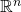). Previously we saw that the plain Gradient Descent algorithm has a rate of convergence of orderaftersteps, while the lower bound that we proved is of order.

We present now a beautiful algorithm due to Nesterov, called Nesterov’s Accelerated Gradient Descent, which attains a rate of order. First we define the following sequences:(Note that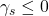.) Now the algorithm is simply defined by the following equations, with an arbitrary initial point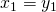,In other words, Nesterov’s Accelerated Gradient Descent performs a simple step of gradient descent to go fromto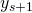, and then it ‘slides’ a little bit further thanin the direction given by the previous point.

The intuition behind the algorithm is quite difficult to grasp, and unfortunately the analysis will not be very enlightening either. Nonetheless Nesterov’s Accelerated Gradient is an optimal method (in terms of oracle complexity) for smooth convex optimization, as shown by the following theorem. [Added in September 2015: we now have a simple geometric explanation of the phenomenon of acceleration, see this post.]

Theorem (Nesterov 1983)
Letbe a convex and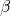-smooth function, then Nesterov’s Accelerated Gradient Descent satisfiesWe follow here the proof by Beck and Teboulle from the paper ‘A fast iterative shrinkage-thresholding algorithm for linear inverse problems‘.

Proof: We start with the following observation, that makes use of Lemma 1 and Lemma 2 from the previous lecture: let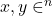, thenNow let us apply this inequality toand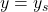, which gives

(1)Similarly we apply it toand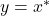which gives

(2)Now multiplying (1) byand adding the result to (2), one obtains with,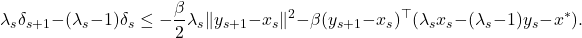Multiplying this inequality byand using that by definitionone obtains

(3)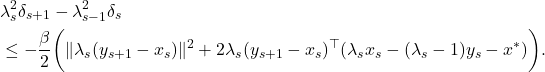Now one can verify that

(4)Next remark that, by definition, one has

(5)Putting together (3), (4) and (5) one gets with,Summing these inequalities fromtoone obtains:By induction it is easy to see thatwhich concludes the proof.This entry was posted in Optimization. Bookmark the permalink.

## 13 Responses to "ORF523: Nesterov’s Accelerated Gradient Descent"

•••••